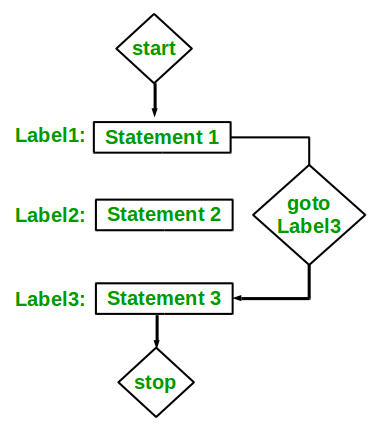Related Articles

# Sum of array Elements without using loops and recursion

• Difficulty Level : Easy
• Last Updated : 09 Apr, 2021

Given an array of N elements, the task is to find the Sum of N elements without using loops(for, while & doWhile) and recursion.
Examples:

```Input: arr[]={1, 2, 3, 4, 5}
Output: 15

Input: arr[]={10, 20, 30}
Output: 60```

Approach: Unconditional Jump Statements can be used to solve this problem.
Unconditional Jump Statements:
Jump statements interrupt the sequential execution of statements, so that execution continues at a different point in the program. A jump destroys automatic variables if the jump destination is outside their scope. There are four statements that cause unconditional jumps in C: break, continue, goto, and return.
To solve this particular problem, goto statement can be useful.
goto Statement:
The goto statement is a jump statement which is sometimes also referred to as unconditional jump statement. The goto statement can be used to jump from anywhere to anywhere within a function.
Syntax

```Syntax1      |   Syntax2
----------------------------
goto label;  |    label:
.                  |    .
.                  |    .
.                  |    .
label:          |    goto label;```

In the above syntax, the first line tells the compiler to go to or jump to the statement marked as a label. Here label is a user-defined identifier which indicates the target statement. The statement immediately followed after ‘label:’ is the destination statement. The ‘label:’ can also appear before the ‘goto label;’ statement in the above syntax.Below is the implementation of the above approach:

## C++

 `// C++ program to find the sum of``// N elements with goto statement` `#include ``using` `namespace` `std;` `// Function to perform desired operation``int` `operate(``int` `array[], ``int` `N)``{``    ``int` `sum = 0, index = 0;` `label:``    ``sum += array[index++];` `    ``if` `(index < N) {` `        ``// backward jump of goto statement``        ``goto` `label;``    ``}` `    ``// return the sum``    ``return` `sum;``}` `// Driver Code``int` `main()``{` `    ``// Get N``    ``int` `N = 5, sum = 0;` `    ``// Input values of an array``    ``int` `array[] = { 1, 2, 3, 4, 5 };` `    ``// Find the sum``    ``sum = operate(array, N);` `    ``// Print the sum``    ``cout << sum;``}`

## C

 `// C program to find the sum of``// N elements with goto statement` `#include ` `// Function to perform desired operation``int` `operate(``int` `array[], ``int` `N)``{``    ``int` `sum = 0, index = 0;` `label:``    ``sum += array[index++];` `    ``if` `(index < N) {` `        ``// backward jump of goto statement``        ``goto` `label;``    ``}` `    ``// return the sum``    ``return` `sum;``}` `// Driver Code``int` `main()``{` `    ``// Get N``    ``int` `N = 5, sum = 0;` `    ``// Input values of an array``    ``int` `array[] = { 1, 2, 3, 4, 5 };` `    ``// Find the sum``    ``sum = operate(array, N);` `    ``// Print the sum``    ``printf``(``"%d"``, sum);``}`

## Java

 `// Java program to find the sum of``// N elements``class` `GFG``{``  ``// Function to perform desired operation``  ``static` `int` `operate(``int` `array[], ``int` `N)``  ``{``    ``int` `sum = ``0``, index = ``0``;      ``    ``while``(``true``)``    ``{``      ``sum += array[index++];      ``      ``if` `(index < N)``      ``{` `        ``// backward jump of goto statement``        ``continue``;``      ``}``      ``else``      ``{``        ``break``;``      ``}``    ``}` `    ``// return the sum``    ``return` `sum;``  ``}` `  ``// Driver code``  ``public` `static` `void` `main(String[] args)``  ``{` `    ``// Get N``    ``int` `N = ``5``, sum = ``0``;` `    ``// Input values of an array``    ``int` `array[] = { ``1``, ``2``, ``3``, ``4``, ``5` `};` `    ``// Find the sum``    ``sum = operate(array, N);` `    ``// Print the sum``    ``System.out.print(sum);``  ``}``}` `// This code is contributed by divyeshrabaiya07`

## Python3

 `# Python3 program to find the sum of``# N elements` `# Function to perform desired operation``def` `operate(array, N) :  ``    ``Sum``, index ``=` `0``, ``0`  `    ``while``(``True``) :``        ``Sum` `+``=` `array[index]``        ``index ``+``=` `1``        ``if` `index < N :``          ` `            ``# backward jump of goto statement``            ``continue``        ``else` `:``            ``break``        ` `    ``# return the sum``    ``return` `Sum``    ` `# Get N``N, ``Sum` `=` `5``, ``0` `# Input values of an array``array ``=` `[ ``1``, ``2``, ``3``, ``4``, ``5` `]` `# Find the sum``Sum` `=` `operate(array, N)` `# Print the sum``print``(``Sum``)` `# This code is contributed by divyesh072019`

## C#

 `// C# program to find the sum of``// N elements with goto statement``using` `System;` `class` `GFG``{``// Function to perform desired operation``static` `int` `operate(``int``[] array, ``int` `N)``{``    ``int` `sum = 0, index = 0;` `label:``    ``sum += array[index++];` `    ``if` `(index < N)``    ``{` `        ``// backward jump of goto statement``        ``goto` `label;``    ``}` `    ``// return the sum``    ``return` `sum;``}` `// Driver Code``public` `static` `void` `Main()``{` `    ``// Get N``    ``int` `N = 5, sum = 0;` `    ``// Input values of an array``    ``int``[] array = { 1, 2, 3, 4, 5 };` `    ``// Find the sum``    ``sum = operate(array, N);` `    ``// Print the sum``    ``Console.Write(sum);``}``}` `// This code is contributed``// by Akanksha Rai`

## PHP

 ``

## Javascript

 ``
Output:
`15`

Attention reader! All those who say programming isn’t for kids, just haven’t met the right mentors yet. Join the  Demo Class for First Step to Coding Coursespecifically designed for students of class 8 to 12.

The students will get to learn more about the world of programming in these free classes which will definitely help them in making a wise career choice in the future.

My Personal Notes arrow_drop_up